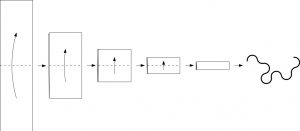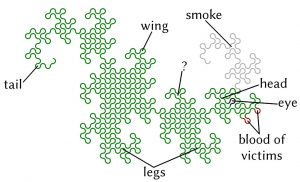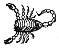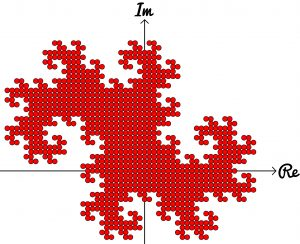# On the cover: dragon curves

Read more about the fire-breathing curves that appear on the cover of issue 05Take a long strip of paper. Fold it in half in the same direction a few times. Unfold it and look at the shape the edge of the paper makes. If you folded the paper $n$ times, then the edge will make an order $n$ dragon curve, so called because it faintly resembles a dragon. Each of the curves shown on the cover of issue 05 of Chalkdust, and in the header box above, is an order 10 dragon curve.Left: Folding a strip of paper in half four times leads to an order four dragon curve (after rounding the corners). Right: A level 10 dragon curve resembling a dragon

The dragon curves on the cover show that it is possible to tile the entire plane with copies of dragon curves of the same order. If any readers are looking for an excellent way to tile a bathroom, I recommend getting some dragon curve-shaped tiles made.

An order $n$ dragon curve can be made by joining two order $n-1$ dragon curves with a 90° angle between their tails. Therefore, by taking the cover’s tiling of the plane with order 10 dragon curves, we may join them into pairs to get a tiling with order 11 dragon curves. We could repeat this to get tilings with order 12, 13, and so on… If we were to repeat this ad infinitum we would arrive at the conclusion that an order $\infty$ dragon curve will cover the entire plane without crossing itself. In other words, an order $\infty$ dragon curve is a space-filling curve.The endpoints of dragon curves of order 1 to 10 with a logarithmic spiral passing through them

Like so many other interesting bits of recreational maths, dragon curves were popularised by Martin Gardner in one of his Mathematical Games columns in Scientific American. In this column, it was noted that the endpoints of dragon curves of different orders (all starting at the same point) lie on a logarithmic spiral. This can be seen in the diagram to the right.

Although many of their properties have been known for a long time and are well studied, dragon curves continue to appear in new and interesting places. At last year’s Maths Jam conference, Paul Taylor gave a talk about my favourite surprise occurrence of a dragon.

Normally when we write numbers, we write them in base ten, with the digits in the number representing (from right to left) ones, tens, hundreds, thousands, etc. Many readers will be familiar with binary numbers (base two), where the powers of two are used in the place of powers of ten, so the digits represent ones, twos, fours, eights, etc.

In his talk, Paul suggested looking at numbers in base -1+i (where i is the square root of -1; you can find more adventures of i here) using the digits 0 and 1. From right to left, the columns of numbers in this base have values 1, -1+i, -2i, 2+2i, -4, etc. The first 11 numbers in this base are shown below.

 Number in base -1+i Complex number 0 0 1 1 10 -1+i 11 (-1+i)+(1)=i 100 -2i 101 (-2i)+(1)=1-2i 110 (-2i)+(-1+i)=-1-i 111 (-2i)+(-1+i)+(1)=-i 1000 2+2i 1001 (2+2i)+(1)=3+2i 1010 (2+2i)+(-1+i)=1+3iNumbers in base -1+i of ten digits or less plotted on an Argand diagram

Complex numbers are often drawn on an Argand diagram: the real part of the number is plotted on the horizontal axis and the imaginary part on the vertical axis. The diagram to the left shows the numbers of ten digits or less in base -1+i on an Argand diagram. The points form an order 10 dragon curve! In fact, plotting numbers of $n$ digits or less will draw an order $n$ dragon curve.

Brilliantly, we may now use known properties of dragon curves to discover properties of base -1+i. A level $\infty$ dragon curve covers the entire plane without intersecting itself: therefore every Gaussian integer (a number of the form $a+\text{i} b$ where $a$ and $b$ are integers) has a unique representation in base -1+i. The endpoints of dragon curves lie on a logarithmic spiral: therefore numbers of the form $(-1+\text{i})^n$, where $n$ is an integer, lie on a logarithmic spiral in the complex plane.

If you’d like to play with some dragon curves, you can download the Python code used to make the pictures here.Matthew is a postdoctoral researcher at University College London. He hasn’t had time to play Klax since the noughties, but he’s pretty sure that Coke is it!